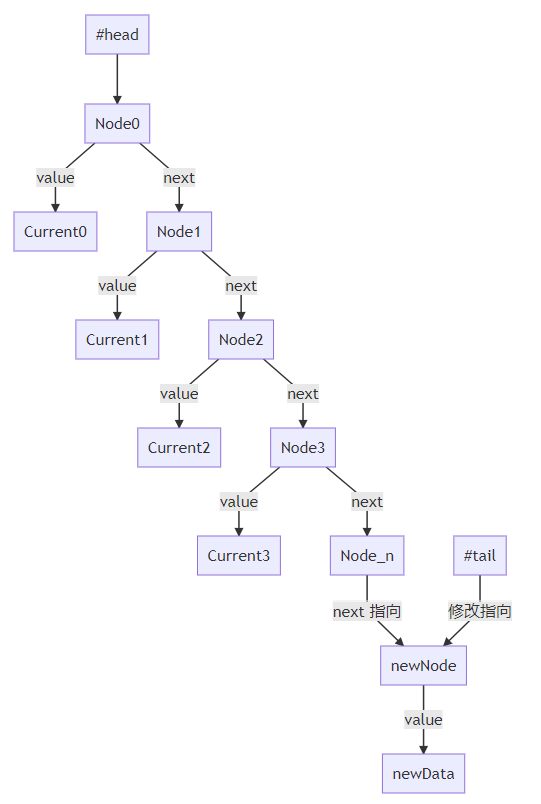﻿ yocto queue微型队列数据结构源码解读_JavaScript_脚本之家
JavaScript# yocto queue微型队列数据结构源码解读

## 引言

`yocto-queue`是一个微型队列的数据结构，根据作者的介绍，如果在你一个数据量很大的数组上，大量的操作`Array.push``Array.shift`，那么你可以考虑使用`yocto-queue`来替代`Array`

## 时间复杂度和空间复杂度

### 时间复杂度

`O`代表的是算法的渐进时间复杂度（asymptotic time complexity），也就是说，随着问题规模的增大，算法的执行时间的增长率和`O`中的函数相同，称作渐进时间复杂度。

`O(1)`表示算法的执行时间与问题规模无关，也就是说，不管问题规模有多大，算法执行所耗费的时间都是一样的，这种算法称为时间复杂度为常数阶的算法。

`O(n)`表示算法的执行时间与问题规模成正比，也就是说，随着问题规模的增大，算法执行所耗费的时间也随之增大，这种算法称为时间复杂度为线性阶的算法。

`O(n^2)`表示算法的执行时间与问题规模成平方比，也就是说，随着问题规模的增大，算法执行所耗费的时间呈二次方增长，这种算法称为时间复杂度为平方阶的算法。

```function multiplyBy2(arr) {
const result = [];
for (let i = 0; i < arr.length; i++) {
result.push(arr[i] * 2);
}
return result;
}
```

```function O(n) {
for (let i = 0; i < n; i++) {
// do something
}
}
O(1); // O(1)
O(2); // O(2)
O(3); // O(3)
O(n); // O(n)
```

### 空间复杂度

```function bubbleSort(arr) {
for (let i = 0; i < arr.length; i++) {
for (let j = 0; j < arr.length - i - 1; j++) {
if (arr[j] > arr[j + 1]) {
const temp = arr[j];
arr[j] = arr[j + 1];
arr[j + 1] = temp;
}
}
}
return arr;
}
```

```function quickSort(arr) {
if (arr.length <= 1) {
return arr;
}
const pivotIndex = Math.floor(arr.length / 2);
const pivot = arr.splice(pivotIndex, 1);
const left = [];
const right = [];
for (let i = 0; i < arr.length; i++) {
if (arr[i] < pivot) {
left.push(arr[i]);
} else {
right.push(arr[i]);
}
}
return quickSort(left).concat([pivot], quickSort(right));
}
```

## 源码分析

### 代码分析

• 安装
```npm install yocto-queue
```
• 使用
```import Queue from 'yocto-queue';
const queue = new Queue();
queue.enqueue('🦄');
queue.enqueue('🌈');
console.log(queue.size);
//=> 2
console.log(...queue);
//=> '🦄 🌈'
console.log(queue.dequeue());
//=> '🦄'
console.log(queue.dequeue());
//=> '🌈'
```

```/*
How it works:
`this.#head` is an instance of `Node` which keeps track of its current value and nests another instance of `Node` that keeps the value that comes after it. When a value is provided to `.enqueue()`, the code needs to iterate through `this.#head`, going deeper and deeper to find the last value. However, iterating through every single item is slow. This problem is solved by saving a reference to the last value as `this.#tail` so that it can reference it to add a new value.
*/
class Node {
value;
next;
constructor(value) {
this.value = value;
}
}
export default class Queue {
#tail;
#size;
constructor() {
this.clear();
}
enqueue(value) {
const node = new Node(value);
this.#tail.next = node;
this.#tail = node;
} else {
this.#tail = node;
}
this.#size++;
}
dequeue() {
if (!current) {
return;
}
this.#size--;
return current.value;
}
clear() {
this.#tail = undefined;
this.#size = 0;
}
get size() {
return this.#size;
}
* [Symbol.iterator]() {
while (current) {
yield current.value;
current = current.next;
}
}
}
```

### 构造函数

```class Queue {
#tail;
#size;
constructor() {
this.clear();
}
}
```

`class`中，如果属性名前面加上`#`，表示这个属性是私有属性，外部是无法访问的；

• `#head`：指向队列的头部；
• `#tail`：指向队列的尾部；
• `#size`：队列的长度；

```class Queue {
clear() {
this.#tail = undefined;
this.#size = 0;
}
}
```

### enqueue

```class Queue {
enqueue(value) {
const node = new Node(value);
this.#tail.next = node;
this.#tail = node;
} else {
this.#tail = node;
}
this.#size++;
}
}
```

`enqueue`方法的作用是向队列中添加一个元素；

```class Node {
value;
next;
constructor(value) {
this.value = value;
}
}
```
• `value`：指向的是队列中的元素；
• `next`：指向下一个`Node`实例；

### dequeue

```class Queue {
dequeue() {
if (!current) {
return;
}
this.#size--;
return current.value;
}
}
```

`dequeue`方法的作用是从队列中移除一个元素；

## 图例`Current0`表示队列中的第一个元素，`Current_n`表示队列中的最后一个元素；`Node0`因为没有任何引用指向它，所以会被垃圾回收机制回收；## 迭代器

`yocto-queue`到最后还提供了一个迭代器，用于遍历队列中的元素；

```class Queue {
// ...
*[Symbol.iterator]() {
while (current) {
yield current.value;
current = current.next;
}
}
}
```

### 迭代器的使用

```const obj = {
[Symbol.iterator]() {
let i = 0;
return {
next() {
return {
value: i++,
done: i > 10
};
}
};
}
};
for (const item of obj) {
console.log(item);
}
```

```const obj = {
*[Symbol.iterator]() {
let i = 0;
while (i < 10) {
yield i++;
}
}
};
for (const item of obj) {
console.log(item);
}
```

Symbol.iterator

Generator 函数

## 总结

`yocto-queue`是一个非常简单的队列实现，它的核心是使用了链表来存储数据；

• 时间复杂度、空间复杂度的概念
• 链表的实现
• 如何使用`es6``Symbol.iterator`来实现可迭代对象；
• 如何使用`es6``generator`函数来实现迭代器；
• 数组、队列、链表的区别；